# Hearts

5 cards are chosen from a standard deck of 52 playing cards (13 hearts) with replacement. What is the probability of choosing 5 hearts in a row?

Correct result:

p =  0.1 %

#### Solution:

$p = 100\cdot \left( \dfrac{13}{52} \right)^{ 5} = 0.1 \%$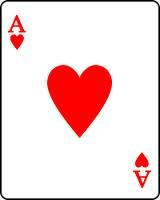We would be pleased if you find an error in the word problem, spelling mistakes, or inaccuracies and send it to us. Thank you!Tips to related online calculators
Would you like to compute count of combinations?

## Next similar math problems:

• BallsFrom the urn in which are 7 white balls and 17 red, gradually drag 3-times without replacement. What is the probability that pulls balls are in order: red red red?
• CardsFrom a set of 32 cards we randomly pull out three cards. What is the probability that it will be seven king and ace?
• Pizza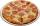A school survey found that 12 out of 15 students like pizza. If 6 students are chosen at random, what is the probability that all 6 students like pizza?
• Two acesFrom a 32 card box we randomly pick 1 card and then 2 more cards. What is the probability that last two drawn cards are aces?
• Components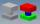In the box are 8 white, 4 blue and 2 red components. What is the probability that we pull one white, one blue and one red component without returning?
• Green - Red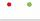We have 5 bags. Each consist one green and 2 red balls. From each we pull just one ball. What is the probability that we doesn't pull any green ball?
• SalamiHow many ways can we choose 5 pcs of salami if we have 6 types of salami for 10 pieces and one type for 4 pieces?
• Events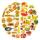Event P has probability of 0.84. What is the probability that the event P occurs in 3, 5, 7 try.
• Hockey players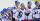After we cycle five hockey players sit down. What is the probability that the two best scorers of this crew will sit next to each other?
• Chambers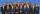The decision-making committee consists of three people. In order for the commission's decision to be valid, at least two members must vote in the same way. It is not possible not to vote in the commission, everyone only votes yes or no. We assume that the
• Probability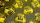What are the chances that the lottery, in which the numbers are drawn 5 of 50 you win the first prize?
• ThrowWe throw 2 times with 2 dices. What is the probability that the first roll will fall more than sum of 9 and the second throw have sum 3 or does not have the sum 4?
• Desks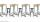A class has 20 students. The classroom consists of 20 desks, with 4 desks in each of 5 different rows. Amy, Bob, Chloe, and David are all friends, and would like to sit in the same row. How many possible seating arrangements are there such that Amy, Bob,
• Raffle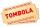There are 200 draws in the raffle, but only 20 of them win. What is the probability of at least 4 winnings for a group of people who have bought 5 tickets together?
• A bookA book contains 524 pages. If it is known that a person will select any one page between the pages numbered 125 and 384, find the probability of choosing the page numbered 252 or 253.
• Boys and girlsThere are eight boys and nine girls in the class. There were six children on the trip from this class. What is the probability that left a) only boys b) just two boys
• Boys and girlsThere are 11 boys and 18 girls in the classroom. Three pupils will answer. What is the probability that two boys will be among them?